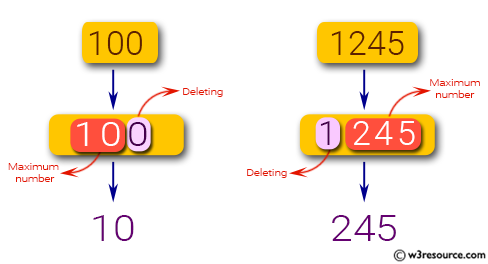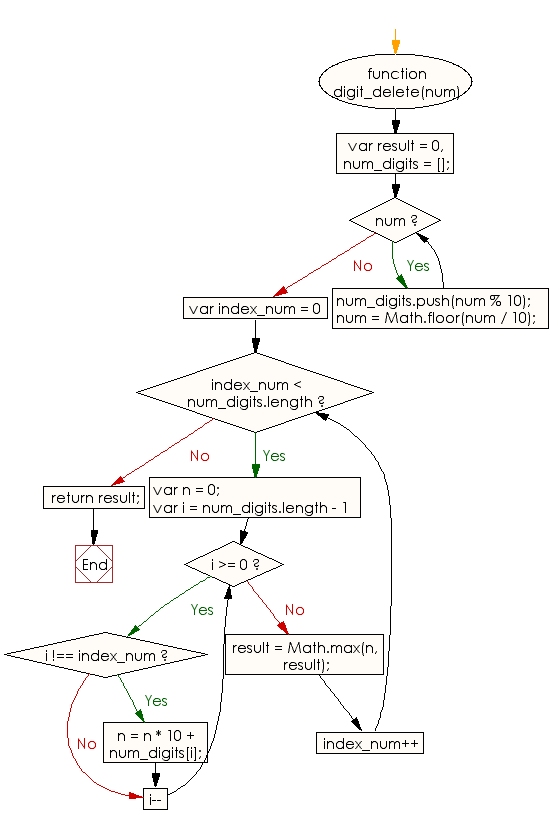# JavaScript: Find the maximum number from a given positive integer by deleting exactly one digit of the given number

## JavaScript Basic: Exercise-103 with Solution

Write a JavaScript program to find the maximum number from a given positive integer by deleting exactly one digit of the given number.

Pictorial Presentation:Sample Solution:

HTML Code:

``````<!DOCTYPE html>
<html>
<meta charset="utf-8">
<meta name="viewport" content="width=device-width">
<title> Find the maximum number from a given positive integer by deleting exactly one digit of the given number</title>
<body>

</body>
</html>
```
```

JavaScript Code:

``````function digit_delete(num) {
var result = 0,
num_digits = [];
while (num) {
num_digits.push(num % 10);
num = Math.floor(num / 10);
}
for (var index_num = 0; index_num < num_digits.length; index_num++) {
var n = 0;
for (var i = num_digits.length - 1; i >= 0; i--) {
if (i !== index_num) {
n = n * 10 + num_digits[i];
}
}
result = Math.max(n, result);
}
return result;
}

console.log(digit_delete(100));
console.log(digit_delete(10));
console.log(digit_delete(1245));
``````

Sample Output:

```10
1
245
```

Flowchart:ES6 Version:

``````function digit_delete(num) {
let result = 0;
const num_digits = [];
while (num) {
num_digits.push(num % 10);
num = Math.floor(num / 10);
}
for (let index_num = 0; index_num < num_digits.length; index_num++) {
let n = 0;
for (let i = num_digits.length - 1; i >= 0; i--) {
if (i !== index_num) {
n = n * 10 + num_digits[i];
}
}
result = Math.max(n, result);
}
return result;
}

console.log(digit_delete(100));
console.log(digit_delete(10));
console.log(digit_delete(1245));
``````

Live Demo:

See the Pen javascript-basic-exercise-103 by w3resource (@w3resource) on CodePen.

What is the difficulty level of this exercise?

Test your Programming skills with w3resource's quiz.

﻿

## JavaScript: Tips of the Day

Converts a string to title case

Example:

```const toTitleCase = str =>
str
.match(/[A-Z]{2,}(?=[A-Z][a-z]+[0-9]*|\b)|[A-Z]?[a-z]+[0-9]*|[A-Z]|[0-9]+/g)
.map(x => x.charAt(0).toUpperCase() + x.slice(1))
.join(' ');
console.log(toTitleCase('some_database_field_name')); // 'Some Database Field Name'
console.log(toTitleCase('Some label that needs to be title-cased')); // 'Some Label That Needs To Be Title Cased'
console.log(toTitleCase('some-package-name')); // 'Some Package Name'
console.log(toTitleCase('some-mixed_string with spaces_underscores-and-hyphens')); // 'Some Mixed String With Spaces Underscores And Hyphens'
```

Output:

```"Some Database Field Name"
"Some Label That Needs To Be Title Cased"
"Some Package Name"
"Some Mixed String With Spaces Underscores And Hyphens"
```